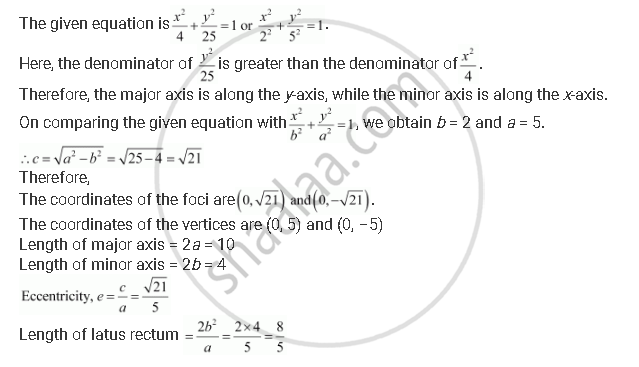Share

# Find the Coordinates of the Foci, the Vertices, the Length of Major Axis, the Minor Axis, the Eccentricity and the Length of the Latus Rectum of the Ellipse X^2/4 + Y^2/25 = 1 - Mathematics

#### Question

Find the coordinates of the foci, the vertices, the length of major axis, the minor axis, the eccentricity and the length of the latus rectum of the ellipse  x^2/4 + y^2/25 = 1

#### SolutionIs there an error in this question or solution?